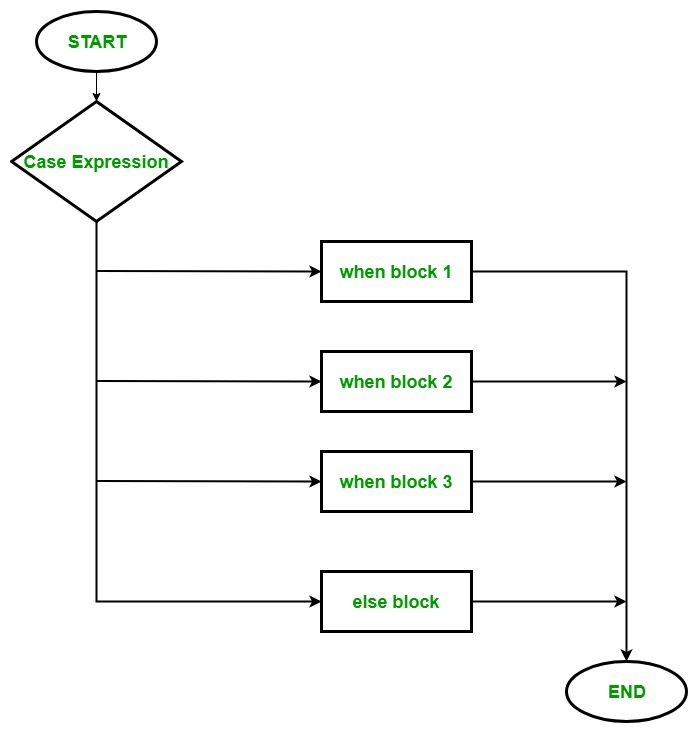GFG App
Open AppBrowser
Continue

The case statement is a multiway branch statement just like a switch statement in other languages. It provides an easy way to forward execution to different parts of code based on the value of the expression.

There are 3 important keywords which are used in the case statement:

• case: It is similar to the switch keyword in another programming languages. It takes the variables that will be used by when keyword.
• when: It is similar to the case keyword in another programming languages. It is used to match a single condition. There can be multiple when statements into a single case statement.
• else: It is similar to the default keyword in another programming languages. It is optional and will execute when nothing matches.

Syntax:

```case expression

when expression 1

when expression 2
.
.

else
end
```

Flow Chart:Example 1:

 `# Ruby program to illustrate the  ` `# concept of case statement ` ` `  `#!/usr/bin/ruby    ` ` `  `print ``"Input from one, two, three, four: "`   ` `  `# taking input from user ` `# str = gets.chomp ` ` `  `# hardcoded input ` `str = ``"two"` ` `  `# using case statement ` `case` `str  ` ` `  `# using when ` `when` `"one"`   `  ``puts ``'Input is 1'` ` `  `when` `"two"`   `  ``puts ``'Input is 2'` ` `  `when` `"three"`   `  ``puts ``'Input is 3'` ` `  ` ``when` `"four"`   `  ``puts ``'Input is 4'` ` `  `else`   `  ``puts ``"Default!"` ` `  `end`

Output:

```Input from one, two, three, four: Input is 2
```

Example 2:

 `# Ruby program to illustrate ` `# case statement ` ` `  `#!/usr/bin/ruby ` ` `  `marks = ``70` ` `  `# marks is the input ` `# for case statement ` `case` `marks ` ` `  `# using range operators .. ` `when` `0``..``32` `  ``puts ``"You fail!"` ` `  `when` `33``..``40` `  ``puts ``"You got C grade!"` ` `  `when` `41``..``60` `  ``puts ``"You got B grade!"` ` `  `else` ` ``puts  ``"You got A grade!"` `  `  `end`

Output:

`You got A grade!`

Important Points:

• In case statement the when statement can contain multiple values and range(see above example).

Example:

 `# Ruby program to illustrate  ` `# how to use multiple values  ` `# in when statement ` ` `  `choice = ``"5"` ` `  `# using 'case' statement ` `case` `choice ` `     `  `    ``# here 'when' statement contains ` `    ``# the two values ` `    ``when` `"1"``,``"2"` `        ``puts ``"You order Espresso!"` `     `  `    ``when` `"3"``,``"4"` `        ``puts ``"You order Short Macchiato!"` `     `  `    ``when` `"5"``,``"6"` `        ``puts ``"You order Ristretto!"` `     `  `    ``when` `"7"``,``"8"` `        ``puts ``"You order Cappuccino!"` `     `  `else` `    ``"No Order!"` `end`

Output:

```You order Ristretto!
```
• You can also use case statement without any value.

Example:

 `# Ruby program to illustrate no  ` `# value in case statement ` ` `  `str = ``"GeeksforGeeks"` ` `  `# here case statement  ` `# has no value ` `case` `     `  `    ``# using match keyword to check ` `    ``when` `str.match(/\d/) ` `          ``puts ``'String contains numbers'` `     `  `    ``when` `str.match(/[a-zA-``Z``]/) ` `          ``puts ``'String contains letters'` `else` `    ``puts ``'String does not contain numbers & letters'` `end`

Output:

`String contains letters`
• You can use case statement in method call. Like method call, a case statement will always return a single object.

Example:

 `# Ruby program to illustrate case  ` `# statement in a method call ` ` `  `str = ``"1234"` ` `  `# here case statement  ` `# has no value & used as  ` `# in puts method call ` `puts ``case` `     `  `    ``# using match keyword to check ` `    ``when` `str.match(/\d/) ` `           `  `        ``'String contains numbers'` `     `  `    ``when` `str.match(/[a-zA-``Z``]/) ` `           `  `        ``'String contains letters'` `else` `     `  `    ``'String does not contain numbers & letters'` `end`

Output:

```String contains numbers
```

Explanation: Here we are using the case statement in a puts method call. The benefit of doing this that we can omit the puts from the when statement.

My Personal Notes arrow_drop_up